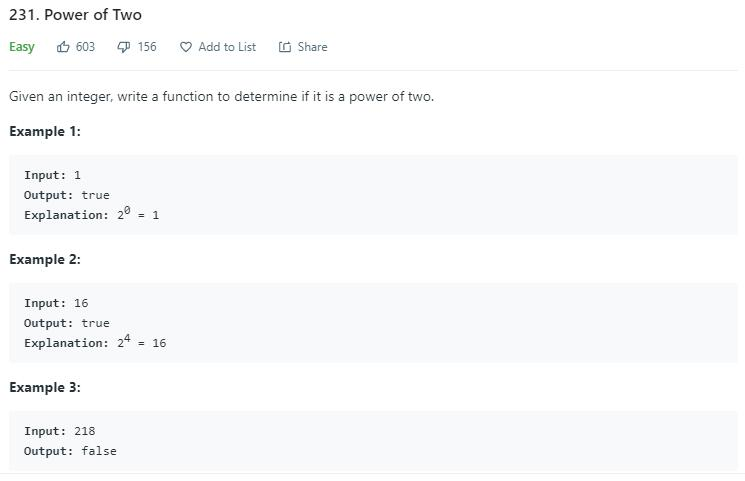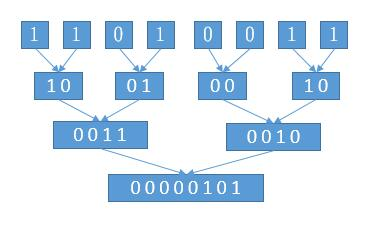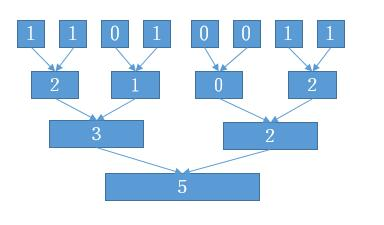# 题目描述（简单难度）# 解法一

public boolean isPowerOfTwo(int n) {
int power = 1;
while (power <= n) {
if (power == n) {
return true;
}
power = power << 1;
// if (power == Integer.MIN_VALUE)
if (power == -2147483648) {
break;
}
}
return false;
}


# 解法二

1 1
2 10
4 100
8 1000
16 10000
...


public boolean isPowerOfTwo(int n) {
if (n == 0 || n == -2147483648) {
return false;
}
return Integer.highestOneBit(n) == n;
}


0-2147483648 不符合上边的规则，单独考虑，我们可以更干脆一些，小于等于 0 的数直接不考虑。

public boolean isPowerOfTwo(int n) {
if (n <= 0) {
return false;
}
return Integer.highestOneBit(n) == n;
}


201 题 的解法三对 highestOneBit 的源码进行了分析，下边把之前的解析贴过来。

/**
* Returns an {@code int} value with at most a single one-bit, in the
* position of the highest-order ("leftmost") one-bit in the specified
* {@code int} value.  Returns zero if the specified value has no
* one-bits in its two's complement binary representation, that is, if it
* is equal to zero.
*
* @param i the value whose highest one bit is to be computed
* @return an {@code int} value with a single one-bit, in the position
*     of the highest-order one-bit in the specified value, or zero if
*     the specified value is itself equal to zero.
* @since 1.5
*/
public static int highestOneBit(int i) {
// HD, Figure 3-1
i |= (i >>  1);
i |= (i >>  2);
i |= (i >>  4);
i |= (i >>  8);
i |= (i >> 16);
return i - (i >>> 1);
}


i       0 0 0 1 1 1 1 1
i >>> 1 0 0 0 0 1 1 1 1
0 0 0 1 0 0 0 0


0 0 0 1 X X X X 变成 0 0 0 1 1 1 1 1，可以通过复制实现。

i |= (i >>  1);
0 0 0 1 X X X X -> 0 0 0 1 1 X X X

i |= (i >>  2);
0 0 0 1 1 X X X -> 0 0 0 1 1 1 1 X

i |= (i >>  4);
0 0 0 1 1 1 1 X -> 0 0 0 1 1 1 1 1



public boolean isPowerOfTwo(int n) {
if (n <= 0) {
return false;
}
int i = n;
i |= (i >> 1);
i |= (i >> 2);
i |= (i >> 4);
i |= (i >> 8);
i |= (i >> 16);
i = i - (i >>> 1);
return i == n;
}


# 解法三

public boolean isPowerOfTwo(int n) {
if (n == 0) return false;
while (n % 2 == 0) {
n /= 2;
}
return n == 1;
}


public boolean isPowerOfTwo(int n) {
return n > 0 && (n == 1 || (n%2 == 0 && isPowerOfTwo(n/2)));
}


# 解法四

public boolean isPowerOfTwo(int n) {
if (n <= 0) {
return false;
}
return (n & (n - 1)) == 0;
}


# 解法五

java 中还有一个方法 Integer.bitCount(n)，返回 n 的二进制形式的 1 的个数。

public boolean isPowerOfTwo(int n) {
if (n <= 0) {
return false;
}
return Integer.bitCount(n) == 1;
}


/**
* Returns the number of one-bits in the two's complement binary
* representation of the specified {@code int} value.  This function is
* sometimes referred to as the <i>population count</i>.
*
* @param i the value whose bits are to be counted
* @return the number of one-bits in the two's complement binary
*     representation of the specified {@code int} value.
* @since 1.5
*/
public static int bitCount(int i) {
// HD, Figure 5-2
i = i - ((i >>> 1) & 0x55555555);
i = (i & 0x33333333) + ((i >>> 2) & 0x33333333);
i = (i + (i >>> 4)) & 0x0f0f0f0f;
i = i + (i >>> 8);
i = i + (i >>> 16);
return i & 0x3f;
}


191 题 题目就是求二进制 1 的个数，其中解法三介绍的其实就是上边的解法，我把解释贴过来。

统计数代表对应括号内 1 的个数
1   1   0   1   0   0   1   1public int hammingWeight(int n) {
n = (n & 0x55555555) + ((n >>> 1) & 0x55555555); // 32 组向 16 组合并，合并前每组 1 个数
n = (n & 0x33333333) + ((n >>> 2) & 0x33333333); // 16 组向 8 组合并，合并前每组 2 个数
n = (n & 0x0f0f0f0f) + ((n >>> 4) & 0x0f0f0f0f); // 8 组向 4 组合并，合并前每组 4 个数
n = (n & 0x00ff00ff)+ ((n >>> 8) & 0x00ff00ff); // 4 组向 2 组合并，合并前每组 8 个数
n = (n & 0x0000ffff) + ((n >>> 16) & 0x0000ffff); // 2 组向 1 组合并，合并前每组 16 个数
return n;
}


public static int bitCount(int i) {
// HD, Figure 5-2
i = i - ((i >>> 1) & 0x55555555);
i = (i & 0x33333333) + ((i >>> 2) & 0x33333333);
i = (i + (i >>> 4)) & 0x0f0f0f0f;
i = i + (i >>> 8);
i = i + (i >>> 16);
return i & 0x3f;
}# 解法六

• 如果 n2 的幂次，那么 $n=2^k$

$2^{30}=2^k*2^{30-k}$，所以 $2^{30} \% 2^k==0$

• 如果 n 不是 2 的幂次，那么 $n = j * 2^k$，其中 j 是一个奇数，因为 n 一直进行除以 2 可以得到 k，直到不能被 2 整除，此时一定是奇数，也就是公式中的 j

$2^{30} \% (j * 2^k) = 2^{30-k} \% j!=0$

public boolean isPowerOfTwo(int n) {
if (n <= 0) {
return false;
}
return 1073741824 % n == 0;
}


# 解法七

public boolean isPowerOfTwo(int n) {
return new HashSet<>(Arrays.asList(1, 2, 4, 8, 16, 32, 64, 128, 256, 512, 1024, 2048, 4096, 8192, 16384, 32768, 65536, 131072, 262144, 524288, 1048576, 2097152, 4194304, 8388608,16777216, 33554432, 67108864, 134217728, 268435456, 536870912, 1073741824)).contains(n);
}